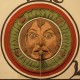# Plate Well Series Plots in R

Plate well series plots are a common way to summarize well level data across multiple plates in a high throughput screen. An example can be seen in Zhang et al. As I’ve been working with RNAi screens, this visualization has been a useful way to summarize screening data and the various transformations on that data. It’s fundamentally a simple scatter plot, with some extra annotations. Though the x-axis is labeled with plate number, the values on the x-axis are actually well locations. The y-axis is usually the signal from that well.

Since I use it often, here’s some code that will generate such a plot. The input is a list of matrices or data.frames, where each matrix or data.frame represents a plate. In addition you need to specify a “plate map” – a character matrix indicating whether a well is a sample, (“c”) positive control (“p”), negative control (“n”) or ignored (“x”). The code looks like

 1234567891011121314151617181920212223242526 plate.well.series <- function(plate.list, plate.map, draw.sep = TRUE, color=TRUE, ...) {   signals <- unlist(lapply(plate.list, as.numeric))   nwell <- prod(dim(plate.list[]))   nplate <- length(signals) / nwell   cols <- 'black'   if (color) {     pcolor <- 'red'     ncolor <- 'green'     colormat <-  matrix(0, nrow=nrow(plate.list[]), ncol=ncol(plate.list[]))     colormat[which(plate.map == 'n')] <- ncolor     colormat[which(plate.map == 'p')] <- pcolor     colormat[which(plate.map == 'c')] <-  'black'     cols <- sapply(1:nwell, function(x) {       as.character(colormat)     })   }   plot(signals, xaxt='n', ylab='Signal', xlab='Plate Number', col = cols, ...)   if (color) legend('topleft', bty='n', fill=c(ncolor, pcolor, 'black'),                     legend=c('Negative', 'Positive', 'Sample'),                     y.intersp=1.2)   if (draw.sep) {     for (i in seq(1, length(signals)+nwell, by=nwell)) abline(v=i, col='grey')   }   axis(side=1, at = seq(1, length(signals), by=nwell) + (nwell/2), labels=1:nplate) }

An example of such a plot is below

Another example comparing normalized data from three runs of an RNAi screen investigating drug sensitization (also highlighting the fact that plate 7 in the 5nm run was messed up):

## 2 thoughts on “Plate Well Series Plots in R”

1.maziz says:

Hi,

Thank you for sharing this code.
I am new to R and trying to run your code but
am unable to do so. Can you please let me know what the format of the plate.list and plate.map should be. I have many 384 well plates with different controls in different locations. How can i get this code to owrk?

Thank you.

2.Rajarshi Guha says:

Hi Maziz, a plate.list is just a list of data.frames, with the 1,1 element representing the top left of the plate. A plate.map is a matrix or data.frame in which all elements are characters. Sample wells are ‘c’, positive and negative controls are ‘p’ and ‘n’ respectively. wells labeled ‘x’ are ignored